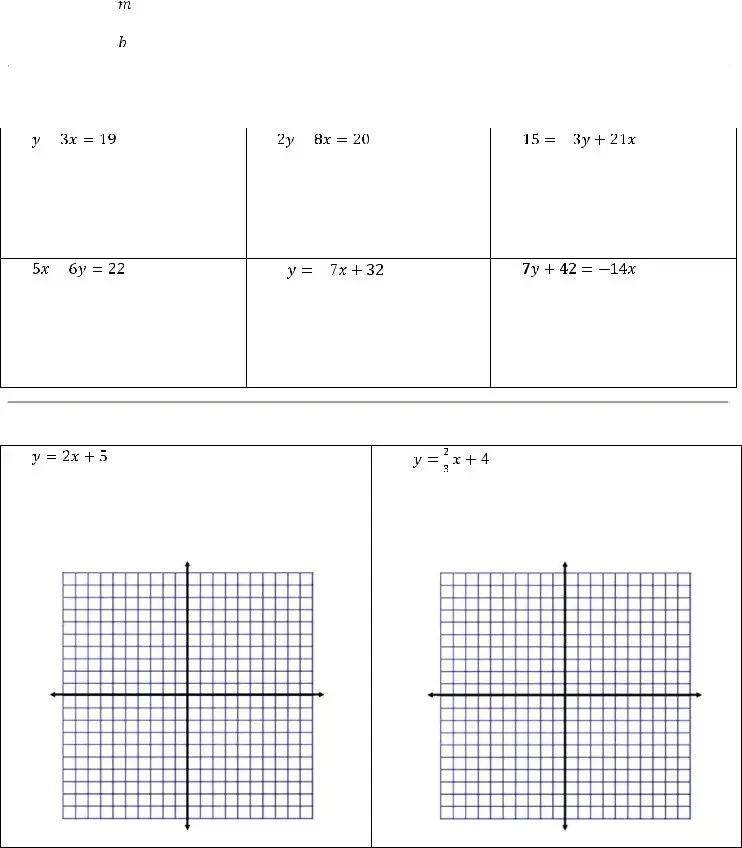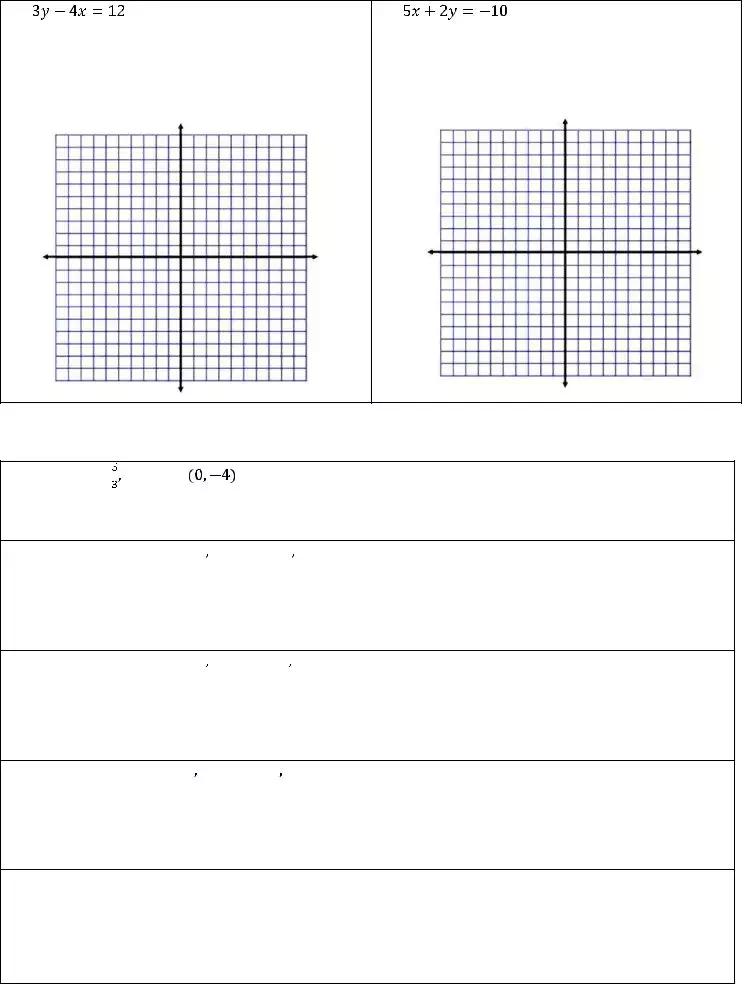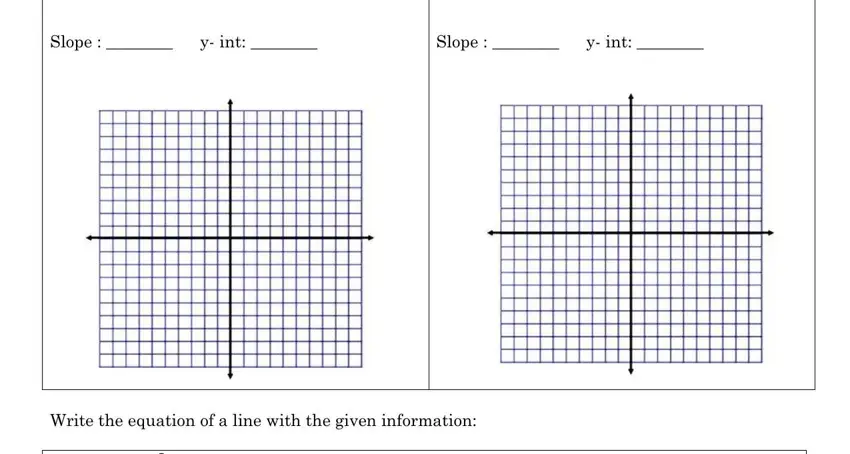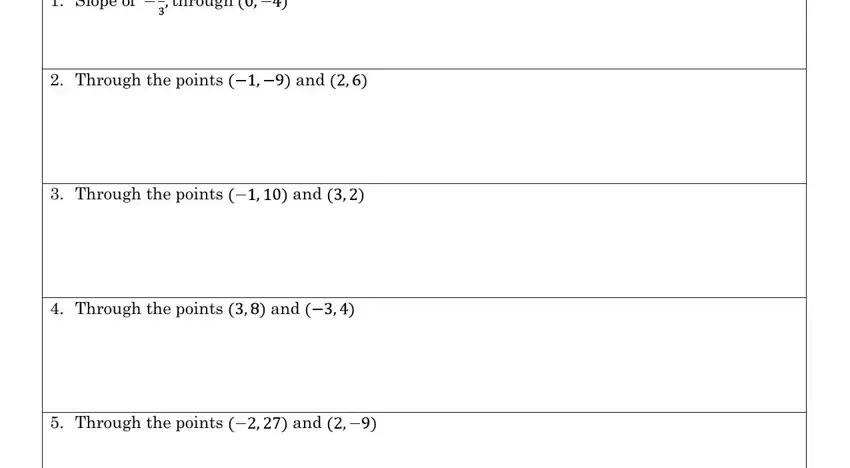## Algebra 1 Slope Intercept Form PDF Details

Algebra 1 slope intercept form is a mathematical equation used to calculate the gradient and y-intercept of a line. The equation can be represented in two ways: as a point (x,y) or as an ordered pair (a,b). In this blog post, we will explore how to use algebra 1 slope intercept form to solve problems and identify lines on graphs. We will also discuss the benefits of using algebra 1 slope intercept form in real world applications.

You will find info about the type of form you would like to fill out in the table. It can show you just how long it will take to fill out algebra 1 slope intercept form, what fields you need to fill in and some other specific details.

Form NameAlgebra 1 Slope Intercept Form
Form Length2 pages
Fillable?Yes
Fillable fields23
Avg. time to fill out5 min 6 sec
Other namesalgebra 1 slope intercept form worksheet 1 answers, slope intercept worksheet 1 algebra form, algebra 1 slope intercept form worksheet, slope intercept worksheet 1 algebra

## Form Preview ExampleAlgebra I Name: __________________________________ Slope Intercept Form Worksheet 1 What is slope intercept form? ___________________________ What does the represent: ______________________ What does the represent: ______________________ Put each equation into slope intercept form. Leave any fractions as simplified improper fractions. 1. 2. 3.
 4. 5. – 6.
 Graph each equation. State the slope and y- intercept. 1. 2. –
 Slope : ________ y- int: ________
 Slope : ________ y- int: ________3. Slope : ________ y- int: ________
 4. Slope : ________ y- int: ________

Write the equation of a line with the given information:

 1. Slope of through

2.Through the pointsand3.Through the pointsand4.Through the pointsand5.Through the pointsand## How to Edit Algebra 1 Slope Intercept Form Online for Free

With the objective of making it as effortless to use as possible, we generated the PDF editor. The entire process of preparing the algebra 1 slope intercept form worksheet 1 answers is going to be simple for those who stick to the next steps.

Step 1: Click the "Get Form Now" button to get started on.

Step 2: Now you will be on the form edit page. You can include, modify, highlight, check, cross, include or remove fields or text.

Fill in the next areas to fill in the form:Write down the information in the Graph each equation, y- in, t , Slope : , and y- in, t area.Outline the necessary information in the y- in, t , and y- in, t field.In the part through, and, and, and and, place the rights and responsibilities of the sides.Fill in the file by looking at the next areas: and.Step 3: As you select the Done button, your ready file can be exported to any of your devices or to email provided by you.

Step 4: Attempt to make as many duplicates of your form as you can to stay away from possible complications.